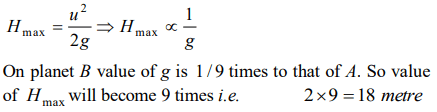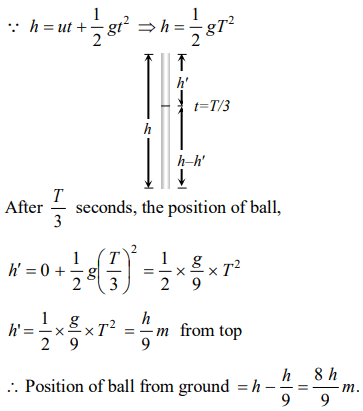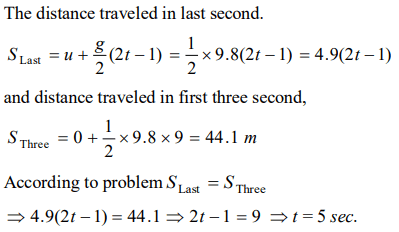## Motion in a Straight Line Questions and Answers Part-19

1. A man drops a ball downside from the roof of a tower of height 400 meters. At the same time another ball is thrown upside with a velocity 50 meter/sec. from the surface of the tower, then they will meet at which height from the surface of the tower
a) 100 meters
b) 320 meters
c) 80 meters
d) 240 meters

Explanation:2. Two balls are dropped from heights h and 2h respectively from the earth surface. The ratio of time of these balls to reach the earth is
a) $1:\sqrt{2}$
b) $\sqrt{2}:1$
c) $2:1$
d) $1:4$

Explanation:3. The acceleration due to gravity on the planet A is 9 times the acceleration due to gravity on planet B. A man jumps to a height of 2m on the surface of A. What is the height of jump by the same person on the planet B
a) 18m
b) 6m
c) $\frac{2}{3}m$
d) $\frac{2}{9}m$

Explanation:4. A body falls from rest in the gravitational field of the earth. The distance travelled in the fifth second of its motion is $\left(g=10 m\diagup s^{2}\right)$
a) 25m
b) 45m
c) 90m
d) 125m

Explanation:5. If a body is thrown up with the velocity of 15 m/s then maximum height attained by the body is $\left(g=10 m\diagup s^{2}\right)$
a) 11.25 m
b) 16.2 m
c) 24.5 m
d) 7.62 m

Explanation:6. A balloon is rising vertically up with a velocity of $\left(g=29 m s^{-1}\right)$    . A stone is dropped from it and it reaches the ground in 10 seconds. The height of the balloon when the stone was dropped from it is $\left(g=9.8 m s^{-2}\right)$
a) 100 m
b) 200 m
c) 400 m
d) 150 m

Explanation:7. A ball is released from the top of a tower of height h meters. It takes T seconds to reach the ground. What is the position of the ball in T/3 seconds
a) h/9 meters from the ground
b) 7h/9 meters from the ground
c) 8h/9 meters from the ground
d) 17h/18 meters from the ground

Explanation:8. Two balls of same size but the density of one is greater than that of the other are dropped from the same height, then which ball will reach the earth first (air resistance is negligible)
a) Heavy ball
b) Light ball
c) Both simultaneously
d) Will depend upon the density of the balls

Explanation: Since acceleration due to gravity is independent of mass, hence time is also independent of mass (or density) of object.

9. A packet is dropped from a balloon which is going upwards with the velocity 12 m/s, the velocity of the packet after 2 seconds will be
a) –12 m/s
b) 12 m/s
c) –7.6 m/s
d) 7.6 m/s